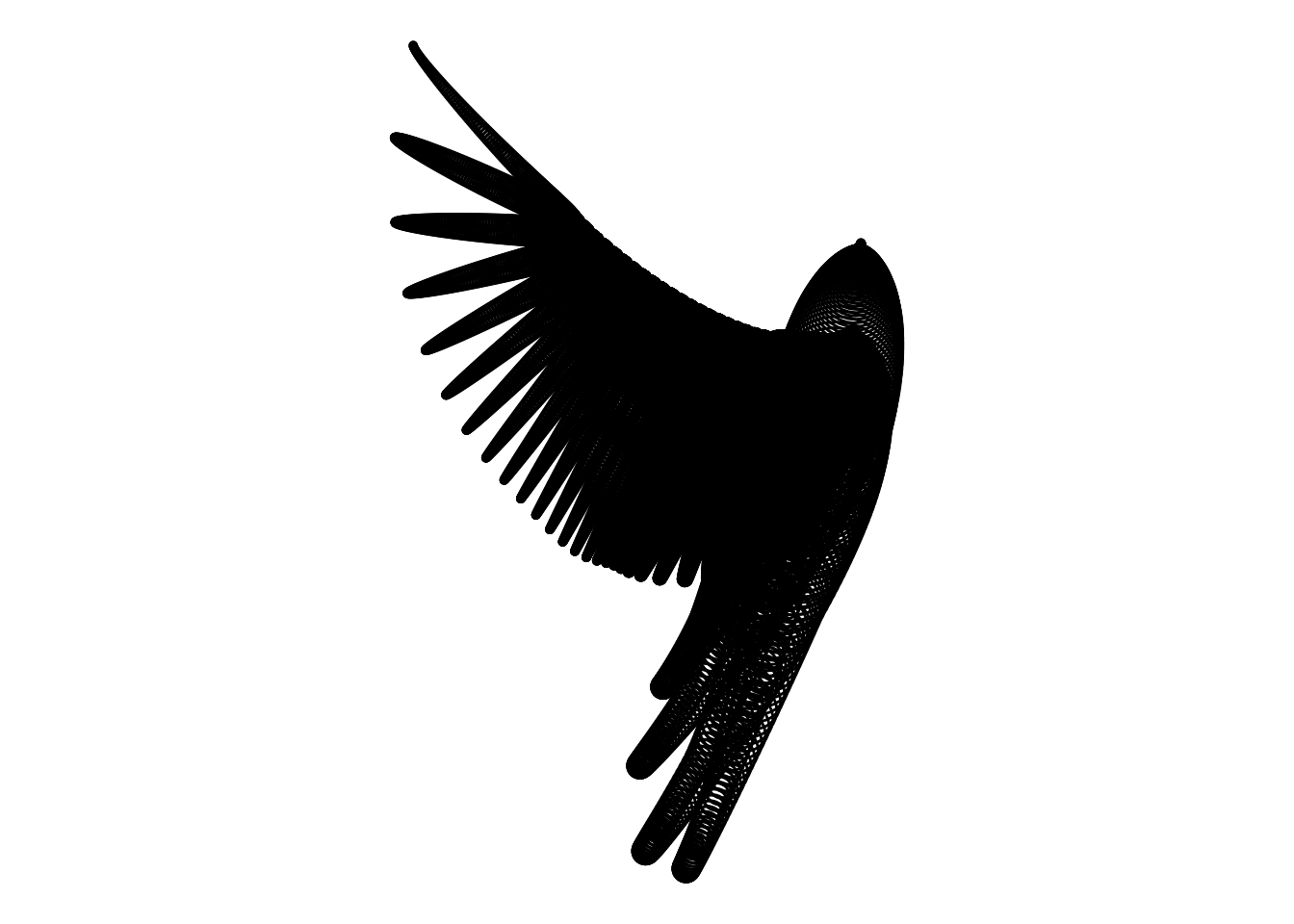# Taking flight with R

## Feb 17, 2018 00:00 · 477 words · 3 minute read R math art visualization

Inspired by the current exhibit on ART ∩ MATH at Seattle’s Center on Contemporary Cart (COCA), I decided to replicate one of the pieces by the very talanted Iranian mathematical artist, Hamid Naderi Yeganeh. Yeganeh provided the full mathematical equation as a caption to his piece, “Bird,” and I thought it would be fun to give it a test flight in R. This turned out to be straightforward thanks to R’s native vectorization:

``````k <- 1:9830

X <- function(k) {
sin(pi * k / 20000) ^ 12 *
(0.5 * cos(31 * pi * k / 10000) ^ 16 *
sin(6 * pi * k / 10000) + (1 / 6 * sin(31 * pi * k / 10000)) ^ 20) +
3 * k / 20000 + cos(31 * pi * k / 10000) ^ 6 *
sin((pi / 2) * ((k - 10000) / 10000) ^ 7 - pi / 5)
}

Y <- function(k) {
-9 / 4 * cos(31 * pi * k / 10000) ^ 6 *
cos(pi / 2 * ((k - 10000) / 10000) ^ 7 - pi / 5) *
(2 / 3 + (sin(pi * k / 20000) * sin(3 * pi * k / 20000)) ^ 6) +
3 / 4 * cos(3 * pi * ((k - 10000) / 100000)) ^ 10 *
cos(9 * pi * ((k - 10000) / 100000)) ^ 10 *
cos(36 * pi * ((k - 10000) / 100000)) ^ 14 +
7 / 10 * ((k - 10000) / 10000) ^ 2
}

R <- function(k) {
sin(pi * k / 20000) ^ 10 *
(1 / 4 * cos(31 * pi * k / 10000 + 25 * pi / 32) ^ 20 +
1 / 20 * cos(31 * pi * k / 10000) ^ 2) +
1 / 30 * (3 / 2 - cos(62 * pi * k / 10000) ^ 2)
}

bird <- data.frame(x = X(k), y = Y(k), r = R(k))``````

Now that we have a data frame of `x` and `y` coordinates, including a vector of radii for the circles centered at these coordinates, we just need to draw the circles. We need to use `coord_fixed()` because each of the 9,830 circles has not only x and y coordinates but a particular radius defined on the same scale. On my first attempt, I used `geom_point` to map the circles’ radii to the size aesthetic. However, the result was distorted because the geom is not scaled 1-to-1 on the coordinate system in which the data is plotted. Fortunately, Thomas Lin Pedersen’s `ggforce` package had exactly the function I needed: `geom_circle`.

``library(ggforce)``
``## Loading required package: ggplot2``
``````p <- ggplot() +
geom_circle(aes(x0 = x, y0 = y, r = r),
data = bird,
n = 30) +
coord_fixed() +
theme_void()
p``````Isn’t math beautiful?

tweet Share